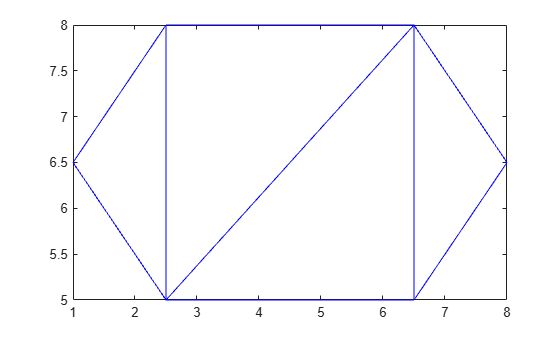# cartesianToBarycentric

Convert coordinates from Cartesian to barycentric

## Syntax

``B = cartesianToBarycentric(TR,ID,C)``

## Description

example

````B = cartesianToBarycentric(TR,ID,C)` returns the barycentric coordinates of the points in `C` relative to the triangulation object `TR`. Each row of `C` contains the Cartesian coordinates of a point with respect to the triangle or tetrahedron indexed by `ID`. The identification numbers of the triangles or tetrahedra in `TR` are the corresponding row numbers of the property `TR.ConnectivityList`.```

## Examples

collapse all

Create a triangulation from a set of points `P` and triangulation connectivity list `T`, and plot the triangulation.

```P = [2.5 8.0; 6.5 8.0; 2.5 5.0; 6.5 5.0; 1.0 6.5; 8.0 6.5]; T = [5 3 1; 3 2 1; 3 4 2; 4 6 2]; TR = triangulation(T,P); triplot(TR)```Find the Cartesian coordinates of the third vertex in the first (leftmost) triangle in `TR`.

```L = TR.ConnectivityList(1,3); C = TR.Points(L,:)```
```C = 1×2 2.5000 8.0000 ```

Convert the point `C` to barycentric coordinates with respect to the first triangle.

`B = cartesianToBarycentric(TR,1,C)`
```B = 1×3 0 0 1 ```

## Input Arguments

collapse all

Triangulation representation, specified as a scalar `triangulation` or `delaunayTriangulation` object.

Data Types: `triangulation` | `delaunayTriangulation`

Triangle or tetrahedron identification, specified as a scalar or a column vector whose elements each correspond to a single triangle or tetrahedron in the triangulation object. The identification number of each triangle or tetrahedron is the corresponding row number of the `ConnectivityList` property.

Data Types: `double`

Cartesian coordinates, specified as a two-column matrix for 2-D coordinates or a three-column matrix for 3-D coordinates.

Data Types: `double`

## Version History

Introduced in R2013a